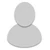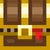## FANDOM

721 Pages

When one actor (Hero/ine, Rat, Goo, etc.) attacks another, two stages take place:

1. test if the attack was successful
2. damage done is calculated

## Test for successful attackEdit

• The attacker generates a random real number between 0 and its accuracy stat, uniformly distributed.
• The defender generates a random real number between 0 and its dodge stat, uniformly distributed.
• If the attacker's number is greater than or equal to the defender's number, the hit is successful.
An example

A level 5 Berserker with strength 15 carrying a +0 War hammer attacks a Gnoll scout:

• The Hero's accuracy depends on his level. At level 5, his accuracy is 14. This accuracy is then multiplied by the weapon's accuracy, which is 1.2, so his accuracy = 14×1.2 = 16.8.
• The Hero generates a real number between 0 and 16.8; lets say he got 8.6
• The Gnoll scout has a dodge stat of 4, so he generates a real number between 0 and 4; lets say he got 2.5.
• Since 8.6 ≥ 2.5, the hit is successful.
Exceptions
• An attack using magic (eg. Wand, Dwarf warlock, Gnoll shaman) will multiply the generated accuracy by 2.
• The Hero/ine's accuracy is decreased when using weapons with a required strength higher than the Hero/ine's strength. The weapons' accuracy is divided by 1.5requiredStrength-heroStrength. In this case, the required strength is 18, and the Hero's strength is 15. So the weapon's accuracy should have been 1.2/(1.518-15) ≈ 0.356; so the Hero's accuracy should have been 14×0.356 ≈ 4.98, instead of 16.8.
In code
  float acuRoll = randomFloat(attacker.accuracy);
float defRoll = randomFloat(defender.dodge);
if (attacker.usingMagic) {
acuRoll *= 2.0F;
}
if (acuRoll >= defRoll) {
// successful hit
} else {
// unsuccessful hit
}
}


## Calculate damageEdit

On a successful hit:

• The attacker generates a random integer between its minimum and maximum hit values, with higher probability to get an integer near the center of the range than near the extrema.
• The defender generates a random integer between 0 and its armor stat.
• The defender takes damage equal to the attackersNumber-defendersNumber, or 0 if the subtraction is negative.
An example

Our level 5 Berserker with strength 15 and +0 War hammer attacks the Gnoll scout:

• The Hero's min/max hit is taken from his weapon which for the War hammer is 5 to 25 (15 ±4.3).
• The Hero generates a random integer between 5 and 25, let's say it equals 18.
• The Gnoll scout has an armor stat of 4, so it generates a damage reduction randomly between 0 and 4; let's say 2.
• The Gnoll scout then takes 18-2=16 damage points. Since the Gnoll scout only has 12 HP, he dies.
Exceptions
• If the Sniper is attacking, then the damage reduction is 0.
• A Furious Berserker damage attack will be multiplied by 1.5 (rounded down if the result is not an integer).
• If the Hero/ine has excess strength when using a weapon, then s/he gains an extra random damage 0 to hero/ineStrength-weaponStrength.
In code
if attacker is Sniper {
absorption = 0;
} else {
absorption = randomInteger(0, defender.armor);
}
damage = max(attacker.damageRoll() - absorption, 0);
// attackProc applies additional effects like debuffs, defenseProc applies resistances and immunities
effectiveDamage = defender.defenseProc(attacker, attacker.attackProc(defender, damage));


## Surprise attackEdit

A surprise attack is an attack that can be made by the Hero/ine or by an enemy. Surprise attacks have 100 % accuracy, even on non-assassin classes.

The Assassin sub-class will get 50 % more damage from surprise attacks.

Surprise attacks can be made if:

• An enemy is sleeping (the Hero/ine can't get surprise-attacked while sleeping because s/he will get a warning if the enemy is in his/her field of view).
• An enemy, or the Hero/ine, has just appeared in the field of view of his/her/its opponent (the one that gets attacked first will be surprise-attacked).
• One instance of that case is the door strategy: Closing a door will make you leave the enemy's field of view, so when that enemy opens the door afterwards, you just appear (again) in its field of view, hence the surprise attack.
• Another instance is when you go around a corner with enough distance from the enemy (if it's just following you, it will still see you all along the way, the Hero/ine won't leave/re-enter its field of view).
• An enemy, or the Hero/ine, is paralyzed with the stunning enchantment/curare dart, even when it is actively hunting the Hero/ine.
• An enemy, or the Hero/ine, is wandering and gets hit (they will get surprise-attacked).

## VisionEdit

FORMULA NEEDED: This is the formula that determines whether or not the Hero/ine can see an enemy. Specifically of interest is seeing around corners.

### TipsEdit

The Scrolls of Psionic Blast, Terror, and Lullaby, and the Huntress Cloak’s Spectral Blades ability, will affect all enemies within your Hero/ine's field of view. When combined with the Potion of Mind Vision, they will affect all enemies currently at that depth.

## TargetingEdit

The code that governs projectiles is Here. Sadly, there is no formula to tell us where to tap in order to hit an enemy at position 42, as it depends on the terrain between you and that enemy. However, for all the sharpshooters out there, a formula exists that shows which tiles are visited along the projectile's flight towards a tile that you tap.

• Imagine you have a rectangle of cells with size (A=16 columns) x (B=9 rows).
 0 1 2 3 4 5 6 7 8 9 10 11 12 13 14 15 16 17 18 19 20 21 22 23 24 25 26 27 28 29 30 31 32 48 64 80 96 112 113 114 115 116 117 118 119 120 121 122 123 124 125 126 127 128 129 130 131 132 133 134 135 136 137 138 139 140 141 142 143
• Cells are labeled from 0 (your position) to 143 (target cell where you tap)
• Cell numbers increase first in the longer axis (rows are longer, so the first row contains cells 0-15, 2nd row contains 16-31 and so on). If columns were longer, then the 1st column would contain cells 0-15 ...
• In this case you will travel through 16 cells Cells(0-15)
• The formula for the i-th cell that you will visit on the way is$\color{green} {Cell(i)=i+A*}\frac{\frac{\text{A-1}}{2}+i*(B-1)}{A-1}$, where "$\color{green}\frac{A}{B}$" means integer division (up to a whole number), e.g.$\color{green}\frac{5}{3}=1$, so you disregard the remainder
• Cell(0)=0+16*((15/2+0*8)/15)=16*(7/15)=16*0=0
• Cell(1)=1+16*((15/2+1*8)/15)=1+16*((7+8)/15)=1+16*(15/15)=1+16=17. This is the 2nd cell on row 2
• ...
• Cell(14)=14+16*((15/2+14*8)/15)=14+16*(119/15)=14+16*7=126
• Cell(15)=15+16*((15/2+15*8)/15)=15+16*(127/15)=15+16*8=143
• If there is an obstacle somewhere along the yellow path, the projectile will stop in the previous yellow cell
• Since  can be hit from different positions behind it, it depends what obstacles there are.

Here's how you can understand the formula intuitively:

• The 1st part "i" gives the column position
• The 2nd part A* .... gives the row position
• A-1 = column distance to target
• B-1 = row distance to target
• An intuitive way to decide when you go to the next row is to start with (A-1)/2 (7) and add B-1 (8)  at every step. Then you see if the result is greater or equal to A-1 (15) - if it is, then you go to the next row and subtract A-1 (15). If it isn't, then you stay on the same row and continue adding B-1 (8) ...
• So the movement above will look like this: (row 1)7 => +8=15=0(row 2) => +8=8 => +8=16=1(row 3) => +8=9 => 17=2(row 4) => +8=10 => +8=18=3(row 5) => +8=11 => +8=19=4(row 6) => +8=12 => +8=20=5(row 7) => +8=13 => +8=21=6(row 8) => +8=14 => +8=22 = 7(row 9) => +8=15=0(row 10) ...
• We can continue and reach rows 10 and bigger. This shows how the projectile continues in the case of a wand.

Here is a table I made while trying to figure out the formula. This shows the path of 15 projectiles, all targeting 15 tiles away from your character, who is at position (1,1):

For all math geeks, all other directions should be obtainable from flipping this table symmetrically with respect to the X, Y axis, or the diagonals, as well as by rotating it by 90°, or by using central symmetry with respect to the middle of the cell (1,1).

How to use the table, someone might ask?

• For example, if you have obstacles at (7,4) and (5,4), you can still hit (8,5) by targeting A, because A goes through (6,4) and (8,5).

It was seen somewhere, that Watabou has stated, that if an enemy is visible, then it can be hit with a ranged weapon or wand by tapping at the appropriate tile; and that, sometimes, the tile with the visible enemy is not the tile you need to tap to hit it.

Actually, it might be impossible to hit the enemy if there isn't enough space behind it(i.e. the map ends right after the enemy so you can't tap further).

Given that you're next to an enemy, you can also tap its image on the right side of the screen to hit it.

Ranged-hitting an enemy positioned just behind a corner: http://i.imgur.com/k5YjhIl.png

## Start a Discussion Discussions about Game mechanics/Attacking

•#### 11 Tips from a Veteran

81 messages
•Note concerning what to do if you find a grim weapon: USE IT.  Especially a grim knuckleduster, because you can punch most enemies at le...
•I wrote:Especially a grim knuckleduster, because you can punch most enemies at least twice before they can punch you once, and this makes it m...
•#### Damage explanation confusing

4 messages
•Hello, not the same Fandom User. Even reading everywhere, the damage stat still remains not clear to me. For instance here: "...
•95.120.9.218 wrote:Hello, not the same Fandom User. Even reading everywhere, the damage stat still remains not clear to me. For instance her...
Community content is available under CC-BY-SA unless otherwise noted.